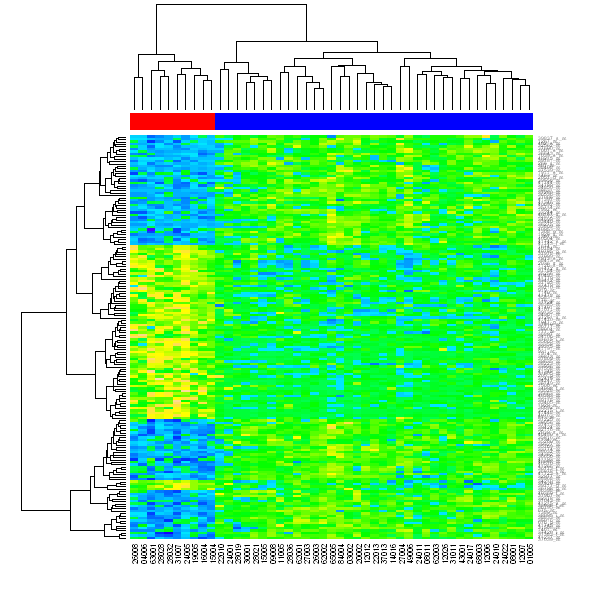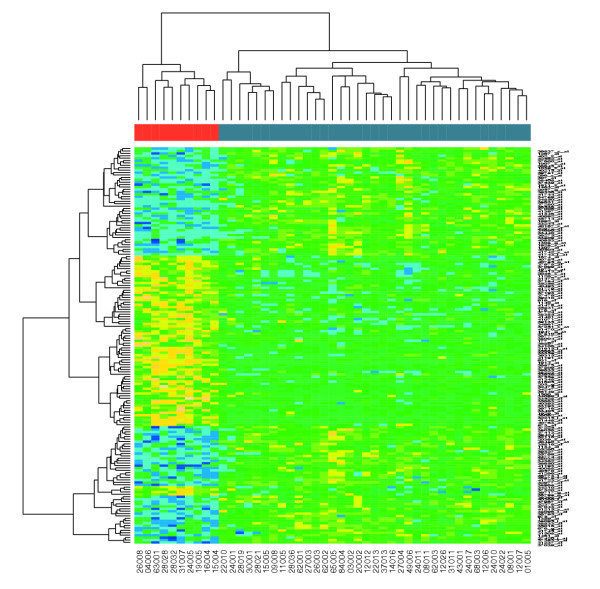# Using Python (and R) to draw a Heatmap from Microarray Data

This document follows on from this page which uses R to analyse an Acute lymphocytic leukemia (ALL) microarray dataset, producing a heatmap (with dendrograms) of genes differentially expressed between two types of leukemia. On this page I deal with how to do this using Python and RPy.

## Before we start...

• a recent version of rpy 1.x (not the new rpy 2.x which would require updating the examples)
• BioConductor
• The acute lymphocytic leukemia dataset, installed as the R ALL package.
I'm also assuming you have read through the example in pure R.

## Heatmaps from Python - Plan (A)

So, how can we do that from within Python? One way is using RPy (R from Python).

We can do the analysis part and the draw the heatmap by calling all the R functions from within Python:

```from rpy import *
set_default_mode(NO_CONVERSION)
r.library("ALL")
r.data("ALL")
r('eset <- ALL[, ALL\$mol.biol %in% c("BCR/ABL", "ALL1/AF4")]')
r.library("limma")
r('f <- factor(as.character(eset\$mol.biol))')
r('design <- model.matrix(~f)')
r('fit <- eBayes(lmFit(eset,design))')
r('selected  <- p.adjust(fit\$p.value[, 2]) < 0.05')
r('esetSel <- eset [selected, ]')
rpy_exprs = r('exprs(esetSel)')

def patient_colour(mol_biol) :
if mol_biol == "ALL1/AF4" :
return "#FF0000" # Red
else :
return "#0000FF" # Blue
#Get r('esetSel\$mol.biol') as a python list...
set_default_mode(BASIC_CONVERSION)
patient_colours = map(patient_colour, r('esetSel\$mol.biol'))

r.heatmap(rpy_exprs,
cexRow=0.5,
ColSideColors = patient_colours,
col = r.topo_colors(50))
```

We end up with this:Again the ColSideColors option is used to assign colours (Red and Blue) to the patients (columns). We are also using the cexRow option to reduce the size of the row captions - otherwise they do overlap each other.

For comparison, the published figure from Gentleman et al. 2004 once again:Tip: If you see a load library error complaining about being unable to load shared library C:\Program Files\R\rw2011\modules\lapack.dll at the lmFit step (on Windows), and you are using rpy-0.4.6 or earlier, make sure that the R bin folder is on the path.

Now while that does work, its a bit of a cheat - its not really using Python for anything other than generating the patient colours list (and I'm sure there is an easy way to do that in R). We have almost literally retyped the R commands, and have kept all the objects in the R format (mode NO_CONVERSION) rather than having RPy translate them into their python equivalents.

## Heatmaps from Python - Plan (B)

So, as an alternative example, we will do the analysis in R, and save the results to a file which we will load from Python. This time we will only be using python data types - so we will be passing heatmap a python array, and the labels to match - rather than an R dataframe with row/column captions built in.

In theory this example could be adapted to show a heatmap of any data you already have accessible to you in existing python programs. Watch out for things like automatic scaling of your data by rows, which may not be appropriate (see the previous section).

From within R, we can use the write.table command to output the expressions table as a file. I'm using a TSV (tab separated variable) file which can be loaded into a spreadsheet like Excel - a blank entry is included using the col.names argument to make the column headers line up properly:

```> write.table(exprs(esetSel),
sep = "\t", quote=FALSE, col.names = NA,
file="all_exprs.tsv")```

You can download the resulting file, Selected ALL expression data file (ALL_exprs.tsv).

We can also export the patients ID (esetSel\$cod) and associated molecular biology results (esetSel\$mol.biol), as just two columns of text:

```>  write.table(cbind(as.character(esetSel\$cod),
as.character(esetSel\$mol.biol)), sep = "\t", quote=FALSE,
col.names = FALSE, row.names=FALSE, file="all_mol_biol.tsv")```

For some reason this strips the leading zeroes off the patient ID's, so I fixed those lines by hand. You can download the resulting file, Selected ALL molecular biology data file (ALL_mol_biol.tsv).

Now we can load these two files in Python, and generate our heatmap. The example script is here: rpy_heatmap.py. The final steps are as follows - notice we are writing graphics to files, rather than just to an onscreen window.

```from rpy import *

print "Heatmap as PNG,",
r.png("heatmap_from_python.png", width=600, height=589)
r.heatmap(exprs_as_array,
cexRow=0.5,
labRow=row_names, labCol=col_names,
ColSideColors = patient_colours,
col = r.topo_colors(50))
r.dev_off()
print "Done"

print "Heatmap as PDF,",
r.pdf("heatmap_from_python.pdf")
r.heatmap(exprs_as_array,
cexRow=0.3,
labRow=row_names, labCol=col_names,
ColSideColors = patient_colours,
col = r.topo_colors(50))
r.dev_off()
print "Done"```

We end up with this PDF figure, and the (same) PNG imageNote that in R, the expression data object contained the row and column names. This isn't possible with python arrays, so we have to use the labRow and labCol options to supply the names separately. Again the ColSideColors option is used to assign colours (Red and Blue) to the patients (columns), and the cexRow option to reduce the size of the row captions to prevent them overlapping each other.

Sample files:

And finally, here is a quick further snippet of code (based on Heatmaps in R - More Options), which uses the heatmap.2 function to include a colour key, and uses the redgreen colour scheme (red to black to green, low to high):

```r.library("gplots")
r.heatmap_2(exprs_as_array,
labRow=row_names,
scale="row",
labCol=col_names,
ColSideColors=patient_colours,
col=r.redgreen(75),
key=True,
symkey=False,
density_info="none",
trace="none",
cexRow=0.5)```

As before, when done purely in R, this yields:This was all written and tested using Windows XP, Python 2.3.3, R-2.1.1 with rpy-0.4.6, and it should all work on other platforms. You may need to adjust the capitalisation of the filenames, and convert them from MS-DOS style newlines to unix style newlines (using dos2unix or something similar). After doing this, the example code also ran perfectly on Ubuntu 5.10, Python 2.4.2, R-2.1.1 with rpy-0.4.3